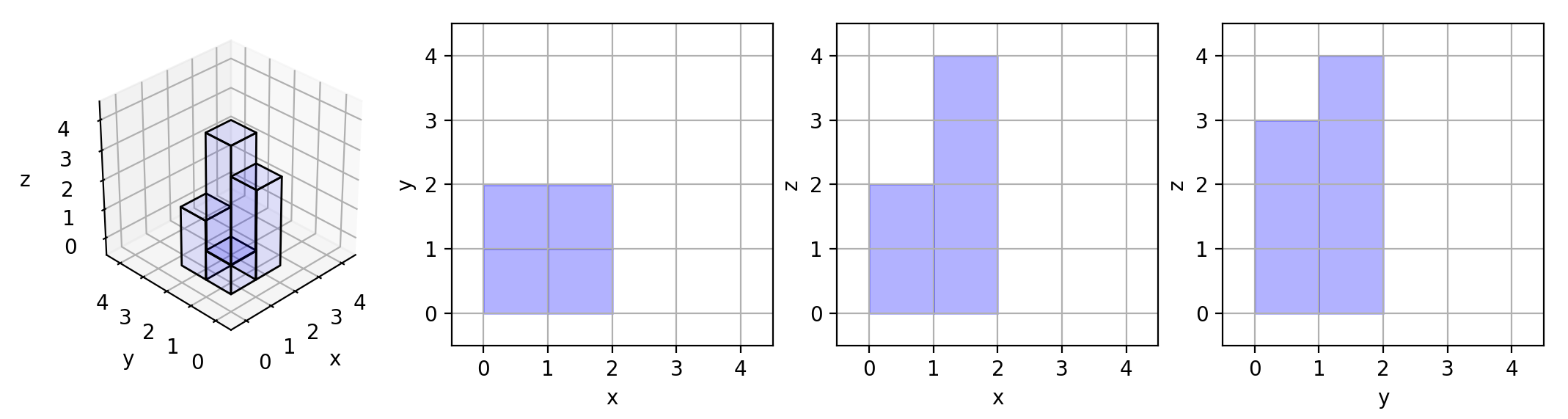# Projection Area of 3D Shapes

Easy

In this lab, you will be working on a problem involving the projection area of 3D shapes. You are given an `n x n` `grid` where we place some `1 x 1 x 1` cubes that are axis-aligned with the `x`, `y`, and `z` axes.

Each value `v = grid[i][j]` represents a tower of `v` cubes placed on top of the cell `(i, j)`.

We view the projection of these cubes onto the `xy`, `yz`, and `zx` planes.

A projection is like a shadow, that maps our 3-dimensional figure to a 2-dimensional plane. We are viewing the "shadow" when looking at the cubes from the top, the front, and the side.

Your task is to create a function that returns the total area of all three projections.

Here are a few examples to clarify the problem:

Example 1:Input: grid = [[1,2],[3,4]] Output: 17 Explanation: Here are the three projections ("shadows") of the shape made with each axis-aligned plane.

Example 2:

Input: grid = [] Output: 5

Example 3:

Input: grid = [[1,0],[0,2]] Output: 8

Constraints:

• `n == grid.length == grid[i].length`
• `1 <= n <= 50`
• `0 <= grid[i][j] <= 50`

To solve this lab, you need to follow the instructions and rewrite the lab material according to the rules specified above.

Challenges:

1. Create a function and name it `projectionArea`. Export this function as ESM.
2. Write test cases for the `projectionArea` function to test various input cases and validate the results.

Evaluation script:

``````1import fs from 'fs'
2import { projectionArea } from '/home/damner/code/index.js'
3
4const testlog = []
5
6try {
7  const result = projectionArea([[1, 2], [3, 4]])
8  if (result === 17) {
9    testlog.push({ status: 'pass' })
10  } else {
11    throw new Error('Incorrect result for example 1')
12  }
13} catch (error) {
14  testlog.push({ status: 'error', error: error.message || 'Challenge failed' })
15}
16
17try {
18  const result = projectionArea([])
19  if (result === 5) {
20    testlog.push({ status: 'pass' })
21  } else {
22    throw new Error('Incorrect result for example 2')
23  }
24} catch (error) {
25  testlog.push({ status: 'error', error: error.message || 'Challenge failed' })
26}
27
28fs.writeFileSync('/home/damner/code/.labtests/testlog.json', JSON.stringify(testlog))
29fs.writeFileSync(process.env.UNIT_TEST_OUTPUT_FILE, JSON.stringify(testlog.map(result => result.status === 'pass')))``````

Adjust the evaluation script accordingly and make sure to complete the missing parts in the lab as per the instructions and rules given. Good luck!